# DataWeave pluck function: How to transform an Object into an Array

We would like to thank MuleSoft Ambassador Joshua Erney for their contribution to this developer tutorial.

In this tutorial, you will learn how to transform an input Object into an Array with the `pluck` function. `pluck` is similar to other Object-like functions, for example, `filterObject` or `mapObject`. However, those two will return an Object, as opposed to `pluck` that returns an Array. You will also learn how to use `pluck` in combination with `groupBy` to achieve a common use case.

You can try all of these examples with the DataWeave Playground. To learn more about it, check out this tutorial.

## Prerequisites

While not required to follow this tutorial, a good understanding of the basic DataWeave concepts would be preferred. You can check out these other tutorials if you feel a bit lost with some concepts:

## Syntax

`pluck` is the function to use if you need to transform an Object into an Array. It takes as inputs an Object and a lambda that accepts 3 parameters: a value (`V`), a key (`K`), and a number representing an index (`Number`). This lambda can return any type (`T`). Whatever type the lambda returns is the same type for each item in the output Array (`Array<T>`).

## Prefix Notation

This function is not commonly used with the prefix notation because of its complexity. But here you can find an example that transforms the input Object into an output Array using all three parameters from the lambda.## Infix Notation

This is the most used notation for this function because it makes it easier to read and understand.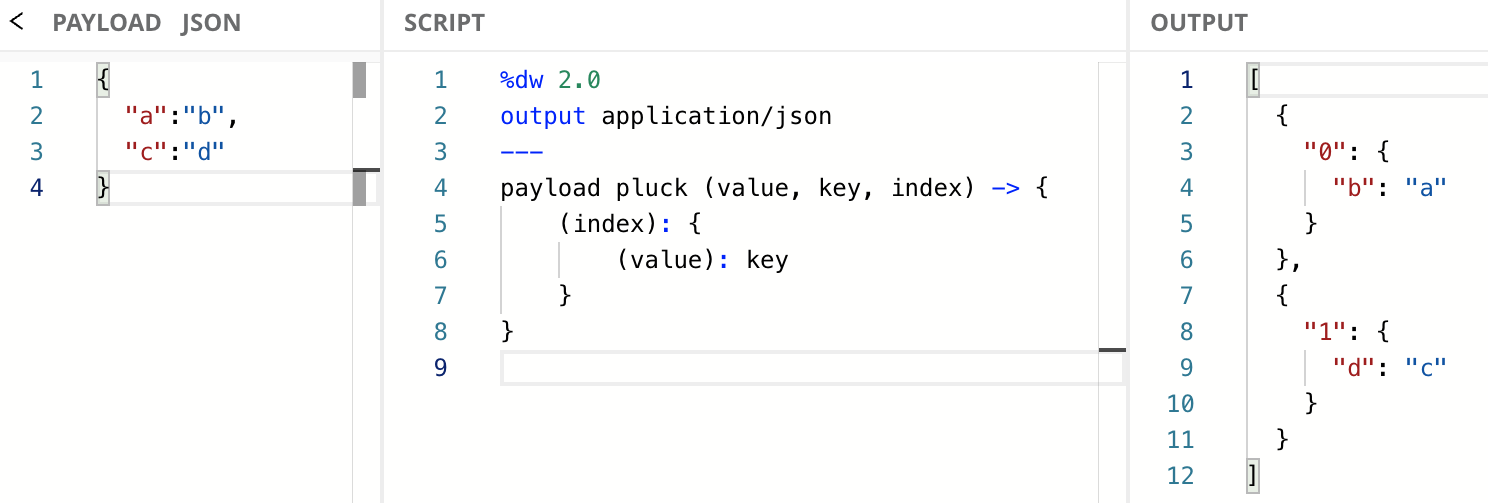When using the infix notation, you don’t have to include all the parameters in the lambda if you’re not using them. However, you do have to include the previous parameters (to the left) whether you’re using them or not. You can only remove the parameters to the right.
In this case, since we are using all 3 parameters, there’s nothing to remove.

## Dollar-sign Syntax

You don’t always need to include the whole explicit lambda expression. You can use the dollar-sign syntax to reference the three arguments that are passed to the lambda (i.e., `value`, `key`, and `index`). `\$` represents the first argument: the value from the key/value pair (`value`), `\$\$` represents the second argument: the key from the key/value pair (`key`), and `\$\$\$` represents the third argument: the index for each key/value pair (`index`).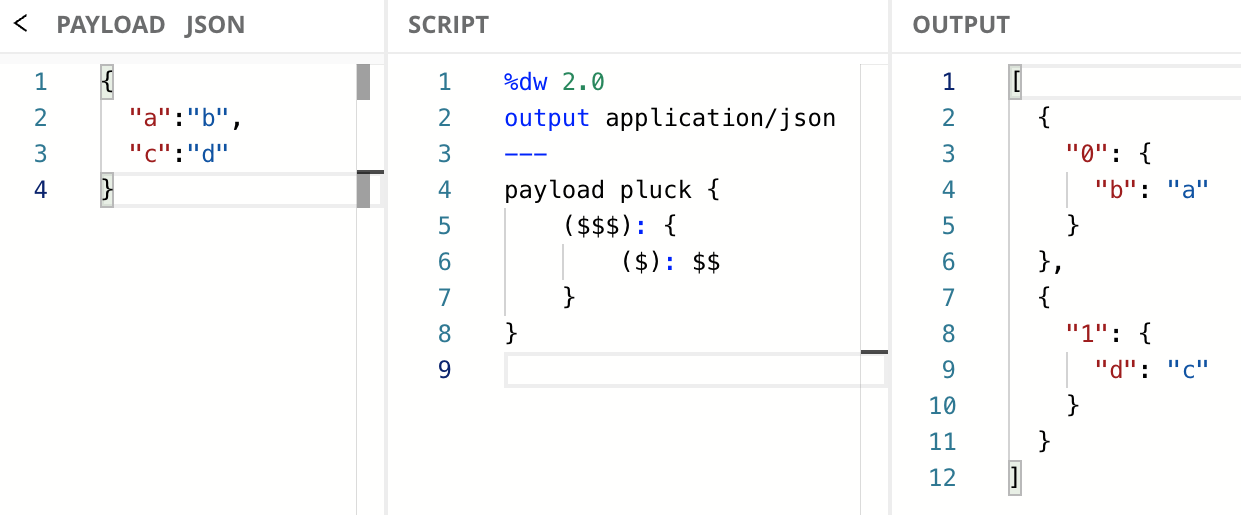## Create an Object per Key/Value Pair

Here’s an example of using `pluck` to take an Object and create an Array where each element is a single key/value pair from the input Object.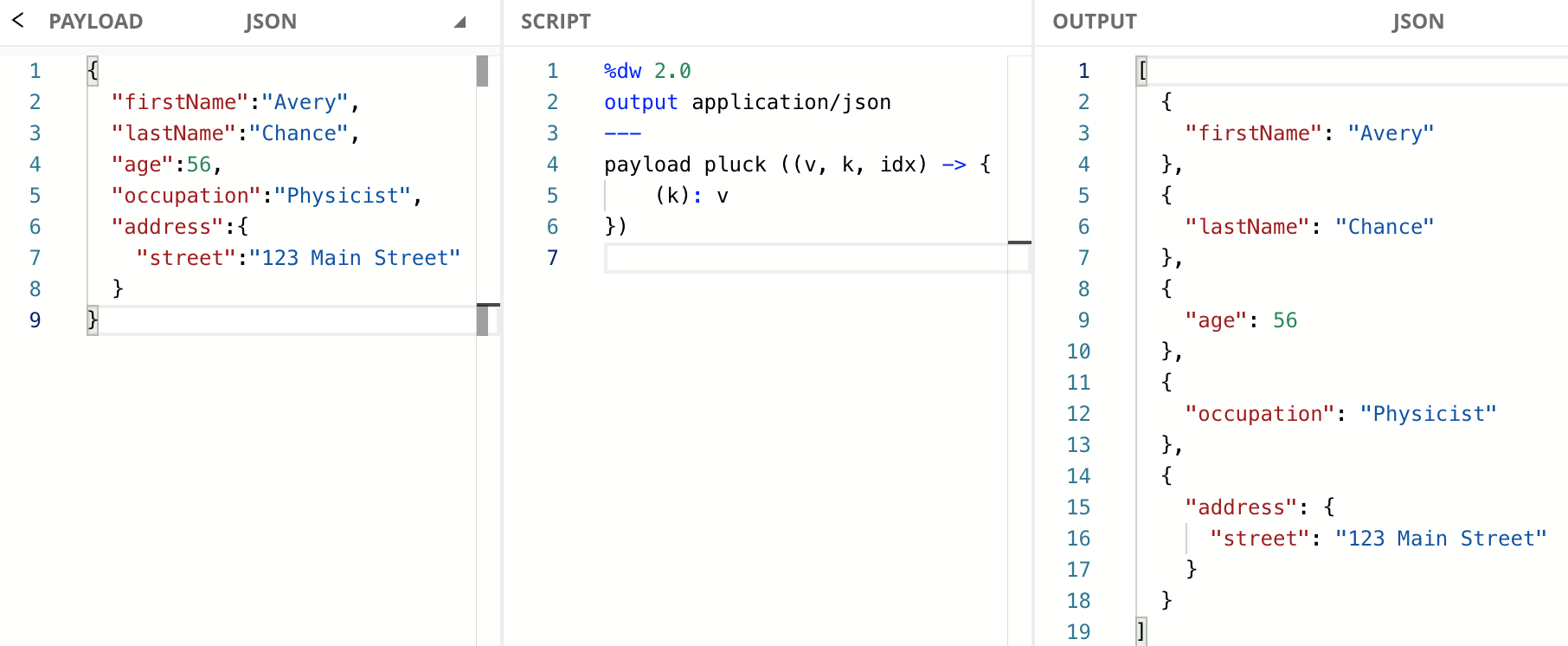When using the infix notation, you don’t have to include all the parameters in the lambda if you’re not using them. In this case, since we’re only using `v` and `k` we can also use the following code:

Finally, here is how the previous code would look like with the dollar-sign syntax:

You need to surround the `k` (or `\$\$`) parameter in parentheses to correctly reference the value of the index when using it as a Key. If you don’t do this, then the String `"k"` will be taken instead.

## Create an Array of Arrays with groupBy

`pluck` is commonly used in conjunction with `groupBy`. This is because oftentimes `groupBy` does exactly what the user wants in terms of grouping data, but the keys labeling the groups are not needed; the user would rather have an Array of Arrays instead of an Object of Arrays. For example, maybe we have a flat representation of multiple product orders and their associated line items.

### Step 1: Group By orderId

The first step is to group the data into their own orders (grouped by `orderId`). Like so: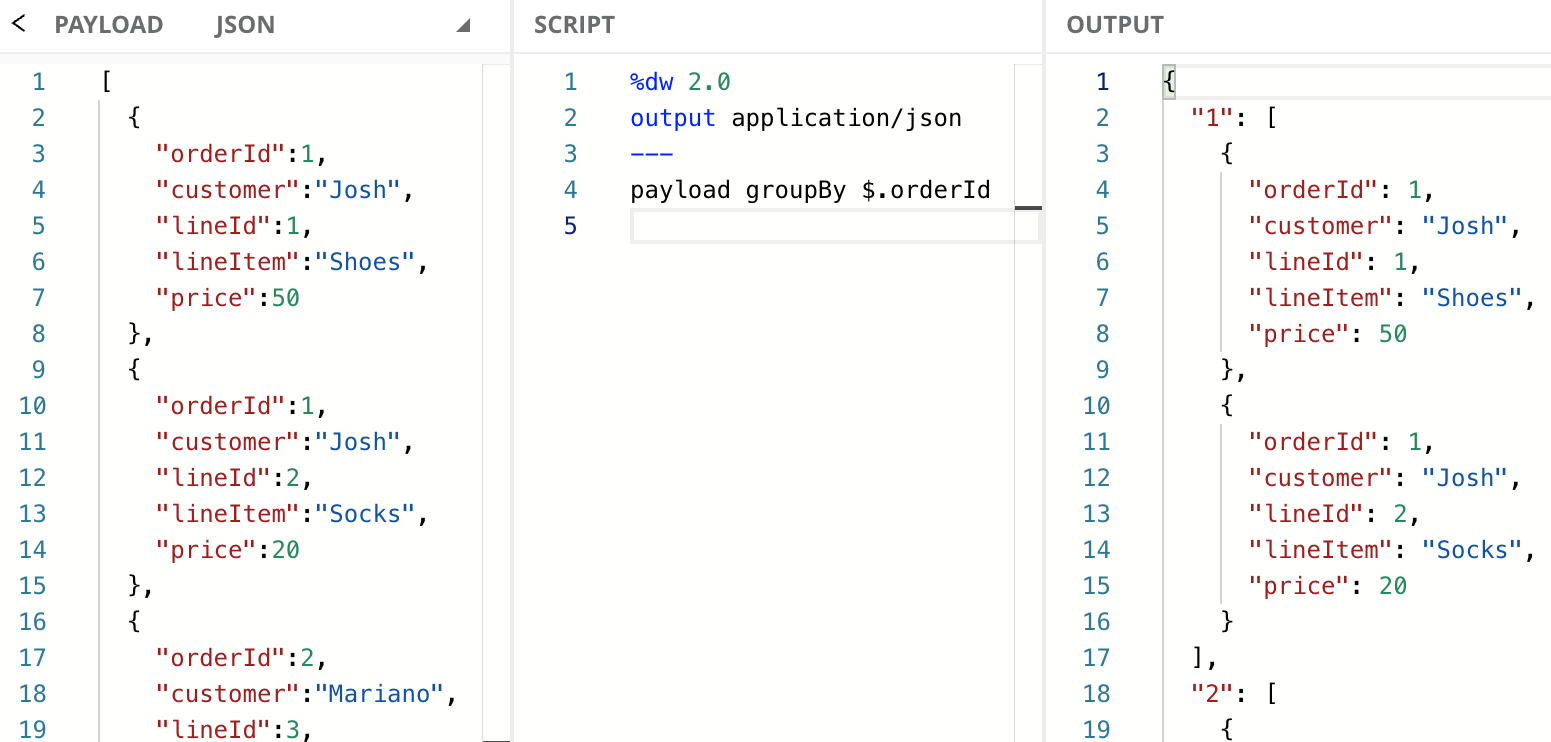If you’re not familiar with the `groupBy` function, it transforms the input Array into an Object with the items grouped in an Array by specific criteria. How to use `groupBy` is out of scope for this tutorial, but you can learn more about it in the documentation or this developer tutorial.

This is great! We’ve effectively grouped all the data into their own orders. If we need to access individual groups and deal with each line individually this is the shape of data we want. However, what if wanted to send the payload into a for-each scope and process each set of order line items individually? We can’t pass an Object to a for-each scope, and we don’t need to label the groups by `orderId` because each item in the group already contains the `orderId`. In this case, we want an Array of Arrays, where each internal Array contains the individual line items for a particular order.

### Step 2: Transform the Object to an Array of Arrays

This is where we use `pluck` after we already grouped the list by `orderId`. We transform the Object that was generated in Step 1 (with its own internal Arrays) into an Array.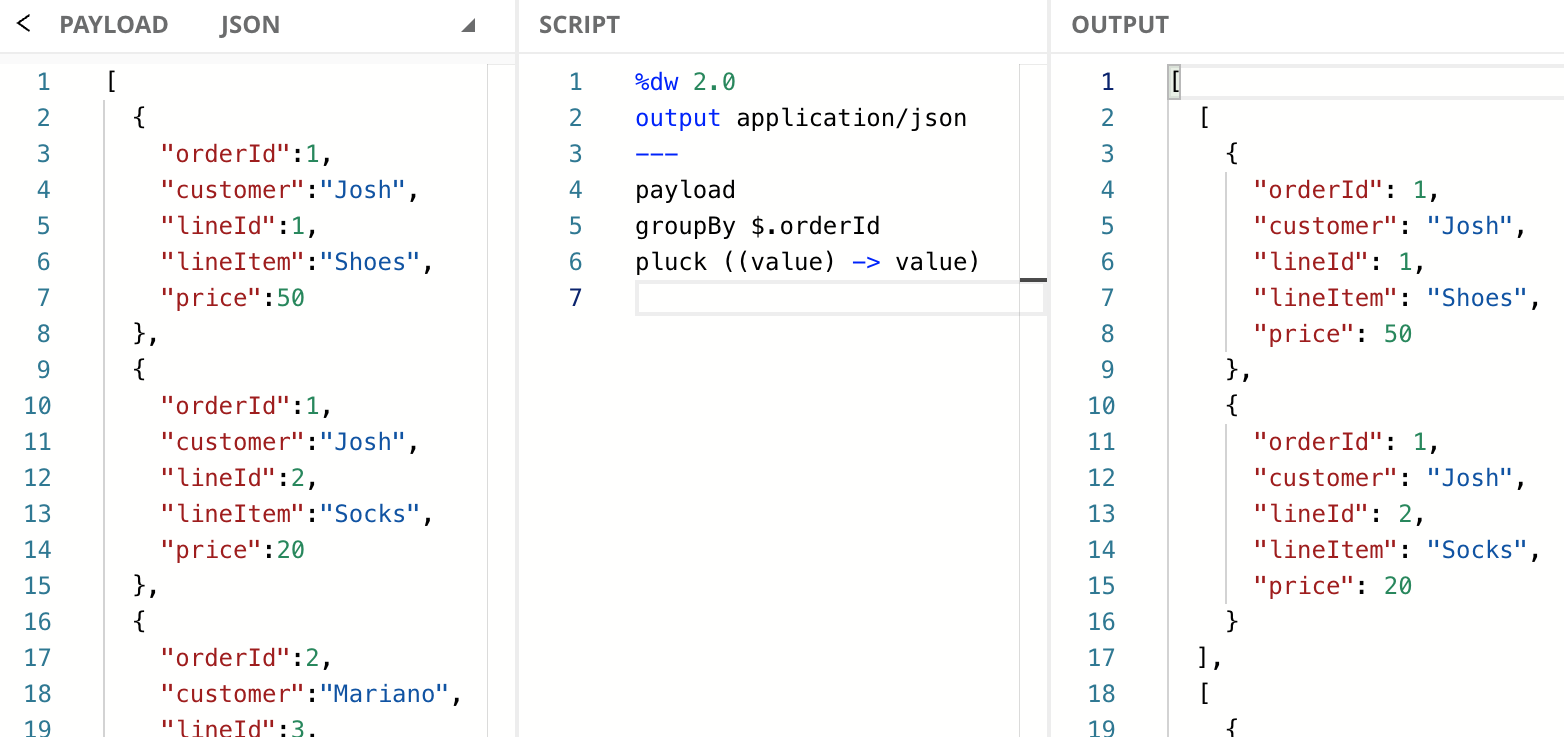Since we’re only using `value` in the `pluck` call from the last line, you can also use the dollar-sign syntax to make the code simpler. Just replace line 6 with this: `pluck \$`

## Nest Steps

In this tutorial, you learned how to transform an input Object into an Array with the `pluck` function. `pluck` is similar to other Object-like functions, for example, `filterObject` or `mapObject`. However, those two will return an Object, as opposed to `pluck` that returns an Array. You also learned how to use `pluck` in combination with `groupBy` to achieve a common use case.

Continue your development journey with the rest of the tutorials to become a master in DataWeave.